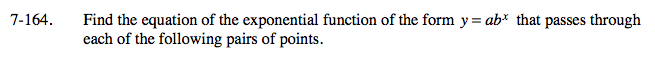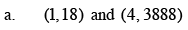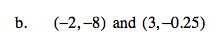### Home > A2C > Chapter 7 > Lesson 7.3.1 > Problem7-164

7-164.Construct two equations by substituting the coordinates
for x and y.

18 = ab1
3888 = ab4

Use substitution to find the value of a and b.

$a = \frac{18}{b}$

$3888 = \frac{18}{b}b^4$

y = 3(6)x

Use the same method as part (a).